NEET  >  Rate Law, Molecularity & Order of a Chemical Reaction

# Rate Law, Molecularity & Order of a Chemical Reaction - NEET

Test Description

## 20 Questions MCQ Test Chemistry Class 12 - Rate Law, Molecularity & Order of a Chemical Reaction

Rate Law, Molecularity & Order of a Chemical Reaction for NEET 2023 is part of Chemistry Class 12 preparation. The Rate Law, Molecularity & Order of a Chemical Reaction questions and answers have been prepared according to the NEET exam syllabus.The Rate Law, Molecularity & Order of a Chemical Reaction MCQs are made for NEET 2023 Exam. Find important definitions, questions, notes, meanings, examples, exercises, MCQs and online tests for Rate Law, Molecularity & Order of a Chemical Reaction below.
Solutions of Rate Law, Molecularity & Order of a Chemical Reaction questions in English are available as part of our Chemistry Class 12 for NEET & Rate Law, Molecularity & Order of a Chemical Reaction solutions in Hindi for Chemistry Class 12 course. Download more important topics, notes, lectures and mock test series for NEET Exam by signing up for free. Attempt Rate Law, Molecularity & Order of a Chemical Reaction | 20 questions in 30 minutes | Mock test for NEET preparation | Free important questions MCQ to study Chemistry Class 12 for NEET Exam | Download free PDF with solutions
 1 Crore+ students have signed up on EduRev. Have you?
Rate Law, Molecularity & Order of a Chemical Reaction - Question 1

### Direction (Q. Nos. 1-13) This section contains multiple choice questions. Each question has four choices (a), (b), (c) and (d), out of which ONLY ONE is correct Q. In the following reaction, which has maximum rate w.r.t. rate of disappearance of NH3? 4NH3 + 502 →  4NO + 6H2O

Detailed Solution for Rate Law, Molecularity & Order of a Chemical Reaction - Question 1

By stoichiometry of the reaction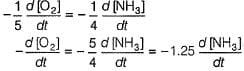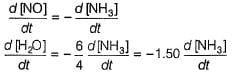Rate Law, Molecularity & Order of a Chemical Reaction - Question 2

### The rate of formation of NO(g) in the reaction, 2NOBr(g) → 2NO(g) + Br2(g) was reported as 1.6 x 10-4 Ms-1. Thus, rate of the reaction is

Detailed Solution for Rate Law, Molecularity & Order of a Chemical Reaction - Question 2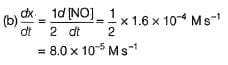Rate Law, Molecularity & Order of a Chemical Reaction - Question 3

### Rate of formation of SO3 in the reaction below is 100 kg min-1. 2SO2 + O2 → 2SO3 Hence, rate of disappearance of SO2 will be:

Detailed Solution for Rate Law, Molecularity & Order of a Chemical Reaction - Question 3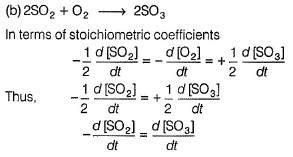Rate of disappearance of SO2 = Rate of formation of SO3 But quantity is to be expressed in terms of moles.
Rate of formation of SO3 = 100 kg SO3 min-1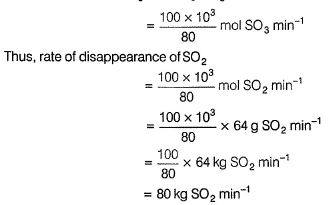Rate Law, Molecularity & Order of a Chemical Reaction - Question 4

The following reaction can take place in both directions: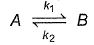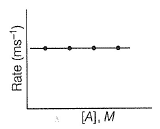For the forward reaction, the rate varies with the concentration of A as and for the backward reaction,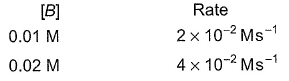Hence, net reaction rate is:

Detailed Solution for Rate Law, Molecularity & Order of a Chemical Reaction - Question 4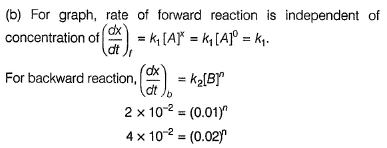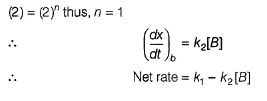Rate Law, Molecularity & Order of a Chemical Reaction - Question 5

For a reaction, xA → yB, rate of disappearance of ‘A’ is related to the rate of appearance of 'B' by the equation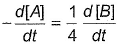Thus, x and y respectively are

Detailed Solution for Rate Law, Molecularity & Order of a Chemical Reaction - Question 5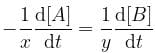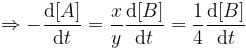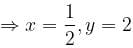Rate Law, Molecularity & Order of a Chemical Reaction - Question 6

For the reaction,

H2(g) + A2(g)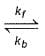2H(g) + A2(g)

kf = 2.2 x 104 L mol-1s-1 and Kc = 1.00 x 10-4 at 3000 K. Thus, kb is

Detailed Solution for Rate Law, Molecularity & Order of a Chemical Reaction - Question 6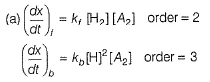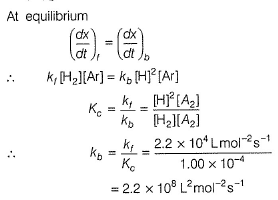Rate Law, Molecularity & Order of a Chemical Reaction - Question 7

For a reaction,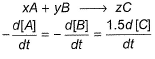hen x, y and z are

Detailed Solution for Rate Law, Molecularity & Order of a Chemical Reaction - Question 7

For the reaction: xA + yB → zC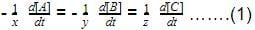Given that,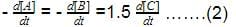Multiplying equation 2 by 1/3, we get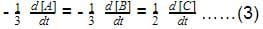On comparing equation 1 and 3,
x = 3, y = 3, z = 2.

Rate Law, Molecularity & Order of a Chemical Reaction - Question 8

For the reaction,

2N2O5(g) → 4NO2 (g) + O2(G)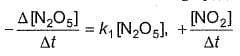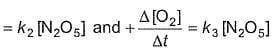Thus,

Detailed Solution for Rate Law, Molecularity & Order of a Chemical Reaction - Question 8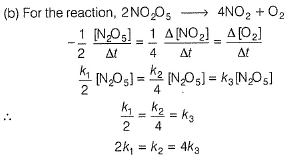Rate Law, Molecularity & Order of a Chemical Reaction - Question 9

The decomposition of acetaldehyde is given by the following reaction:

CH3CHO(g) → CH4(g) + CO(g)

Rate of the reaction with respect to the pressure of the reactant is

Detailed Solution for Rate Law, Molecularity & Order of a Chemical Reaction - Question 9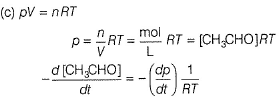Rate Law, Molecularity & Order of a Chemical Reaction - Question 10

For the reaction,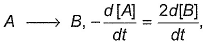then rate law is

Detailed Solution for Rate Law, Molecularity & Order of a Chemical Reaction - Question 10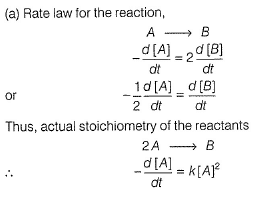Rate Law, Molecularity & Order of a Chemical Reaction - Question 11

For gaseous reaction, the rate can be expressed as

Detailed Solution for Rate Law, Molecularity & Order of a Chemical Reaction - Question 11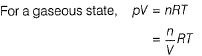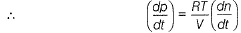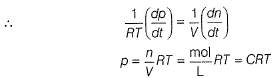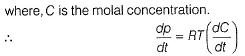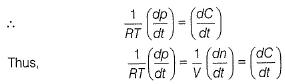Rate Law, Molecularity & Order of a Chemical Reaction - Question 12

The initial rates of reaction for the equation, 2A + B → Products.

Products were determined under various initial concentrations of reactants.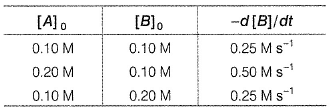Thus, rate law is equal to

Detailed Solution for Rate Law, Molecularity & Order of a Chemical Reaction - Question 12

Let order w.r.t. A = a, order w.r.t. B = b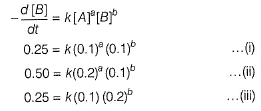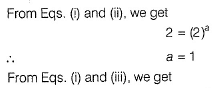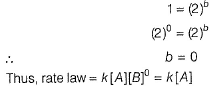Rate Law, Molecularity & Order of a Chemical Reaction - Question 13

For the reaction, A + 2B → Product, the reaction rate was halved on doubling the concentration of A. Thus, order w.r.t. A is

Detailed Solution for Rate Law, Molecularity & Order of a Chemical Reaction - Question 13

If concentration of the nth order reaction is made m time, rate becomes mn times.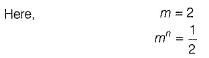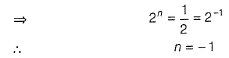Rate Law, Molecularity & Order of a Chemical Reaction - Question 14

Direction (Q. Nos. 14 and 15) This section contains a passage describing theory, experiments, data, etc. Two questions related to the paragraph have been given. Each question has only one correct answer out of the given 4 options (a), (b), (c) and (d)

Passage

The decomposition of NO2 at 400 K proceeds at a of rate of 5.4 x 10 -5 mol L-1 s-1 when [NO2] = 0.01 mol-1

2 NO2(g) → 2NO(g ) + O2(g).

Q. What is the rate law when observed rate is 1.35 x 10-5 mol L-1 s-1 at [NO2] = 0.005 mol L-1?

Detailed Solution for Rate Law, Molecularity & Order of a Chemical Reaction - Question 14

The given information can be summarized as follows:

Decomposition of NO2:
- Reaction: 2 NO2(g) → 2NO(g) + O2(g)
- At 400 K: Rate = 5.4 x 10^(-5) mol L^(-1) s^(-1), [NO2] = 0.01 mol L^(-1)
- Observed rate: 1.35 x 10^(-5) mol L^(-1) s^(-1), [NO2] = 0.005 mol L^(-1)

Let's compare the rates and concentrations given in the problem:

Rate1 = 5.4 x 10^(-5) mol L^(-1) s^(-1), [NO2]1 = 0.01 mol L^(-1)
Rate2 = 1.35 x 10^(-5) mol L^(-1) s^(-1), [NO2]2 = 0.005 mol L^(-1)

Take the ratio of the two rates:

Rate2/Rate1 = (1.35 x 10^(-5))/(5.4 x 10^(-5)) = 1/4

Now, take the ratio of the two concentrations:

[NO2]2/[NO2]1 = (0.005)/(0.01) = 1/2

We can now evaluate each of the rate law options:

a. k[NO2]: (1/2) ≠ 1/4
b. k[NO2]^0: 1 ≠ 1/4
c. k[NO2]^3: (1/2)^3 ≠ 1/4
d. k[NO2]^2: (1/2)^2 = 1/4

Only option (d) satisfies the relationship between the rates and concentrations:
Rate Law:
- k[NO2]^2

Rate Law, Molecularity & Order of a Chemical Reaction - Question 15

The decomposition of NO2 at 400 K proceeds at a of rate of 5.4 x 10 -5 mol L-1 s-1 when [NO2] = 0.01 mol-1

2 NO2(g) → 2NO(g ) + O2(g).

Q. Rate constant of the reaction will be

Detailed Solution for Rate Law, Molecularity & Order of a Chemical Reaction - Question 15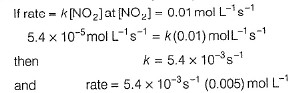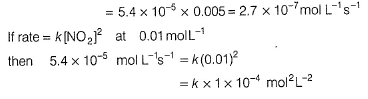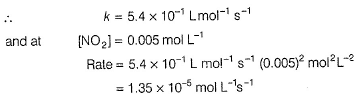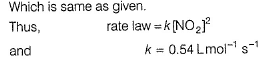Rate Law, Molecularity & Order of a Chemical Reaction - Question 16

Direction (Q. Nos. 16-18) This section contains 3 questions. When worked out will result in one integer from 0 to 9 (both inclusive)

In the following reaction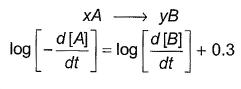Q. Where negative sign indicates rate of disappearance of the reactant. What is the value of x/y ?

Detailed Solution for Rate Law, Molecularity & Order of a Chemical Reaction - Question 16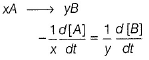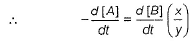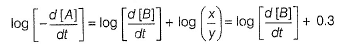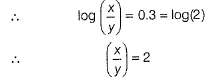Rate Law, Molecularity & Order of a Chemical Reaction - Question 17

The reaction rate is defined as the rate at which the concentration of the reactants __________ with time or the concentration of products ___________ with time.

Detailed Solution for Rate Law, Molecularity & Order of a Chemical Reaction - Question 17

The reaction rate is defined as the rate at which the concentration of the reactants decreases with time or the concentration of products increases with time.

Rate Law, Molecularity & Order of a Chemical Reaction - Question 18

In a certain polluted atmosphere containing O3 at a steady concentration of 2.0 x 10-6 M, the hourly production of O3 by all sources was estimated as 7.2 x 10-15 M. If the only mechanism for destruction of O3 in the second-order reaction is

2O3 → 3O2

then rate constant for destruction reaction, defined by the rate law for - Δ[O3]/Δf is x * 10-7 M-1 s-1 . What is the value of x?

Detailed Solution for Rate Law, Molecularity & Order of a Chemical Reaction - Question 18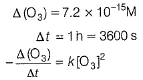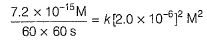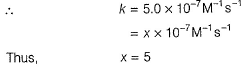Rate Law, Molecularity & Order of a Chemical Reaction - Question 19

Only One Option Correct Type

This section contains 2 multiple choice questions. Each question has four choices (a), (b), (c) and (d), out of which ONLY ONE is correct

For a reversible reaction, net rate is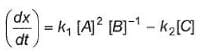hence given reaction is

Detailed Solution for Rate Law, Molecularity & Order of a Chemical Reaction - Question 19

2A - B →(reversible) C

For a forward reaction, (dx/dt)f = k1[A]2 [B]-1
For a backward reaction, (dx/dt)b = k2[C]

Net rate = (dx/dt)f - (dx/dt)= k1[A]2 [B]-1 - k2[C]

Rate Law, Molecularity & Order of a Chemical Reaction - Question 20

Reaction kinetics deals with the study of

Detailed Solution for Rate Law, Molecularity & Order of a Chemical Reaction - Question 20

Reaction kinetics deals with the study of rate of reaction, their mechanism and the factors which affects the rate of reaction. It specifies all the general characteristics of a chemical reaction.

## Chemistry Class 12

150 videos|377 docs|213 tests
Information about Rate Law, Molecularity & Order of a Chemical Reaction Page
In this test you can find the Exam questions for Rate Law, Molecularity & Order of a Chemical Reaction solved & explained in the simplest way possible. Besides giving Questions and answers for Rate Law, Molecularity & Order of a Chemical Reaction, EduRev gives you an ample number of Online tests for practice

## Chemistry Class 12

150 videos|377 docs|213 tests

### How to Prepare for NEET

Read our guide to prepare for NEET which is created by Toppers & the best Teachers